# Coordinate Geometry

Coordinate Geometry
Go back to  'Geometry'

Every place on this planet has coordinates that help us to locate it easily on the world map.

The coordinates of a few important places are as follows:

Boston - $$42.3601^ \circ N$$, $$71.0589 ^\circ W$$

Bengaluru - $$12.9716^ \circ N$$, $$77.5946 ^\circ E$$

Singapore - $$1.3521 ^\circ N$$, $$103.8198 ^\circ E$$

London -  $$51.5074 ^\circ N$$,  $$0.1278 ^\circ W$$

The coordinate system of our earth is made up of imaginary lines called latitudes and longitudes.

The zero degrees 'Greenwich Longitude' and the zero degrees 'Equator Latitude' are the starting lines of this coordinate system.Here, in our study of the coordinate system in math we have the horizontal $$x$$-axis and the vertical $$y$$-axis as the reference lines.

## POINT LOCATOR - Simulation

Let us explore the simulation given below, to gain an initial understanding of the coordinate system.

With the help of your cursor, move the point on the graph and take note of the coordinates of the point on each of the axis, and also in the four quadrants.

In this mini-lesson, we shall explore the topic of coordinate geometry, by finding answers to questions like what is a coordinate plane, what is the difference between a scalar and a vector, and aim to understand the formulae used in coordinate geometry.

## Lesson Plan

 1 What Is a Coordinate? 2 Important Notes on Coordinate Geometry 4 Solved Examples on Coordinate Geometry 3 Challenging Questions on Coordinate Geometry 5 Interactive Questions on Coordinate Geometry

## What Is a Coordinate?

A coordinate is an address, which helps to locate a point in space.

For a two dimensional space, the coordinates of a point are $$(x, y)$$.

Here let us take note of these two important terms.

• Abscicca: It is the $$x$$ value in the point $$(x, y)$$.
• Ordinate:  It is the $$y$$ value in the point $$(x, y)$$.

The $$x$$ coordinate is called the abscissa and the $$y$$ coordinate is called the ordinate.

## What Is a Cartesian Plane Or Coordinate Plane?

A cartesian plane divides the space into two dimensions and is useful to locate the points.

It is also referred to as the coordinate plane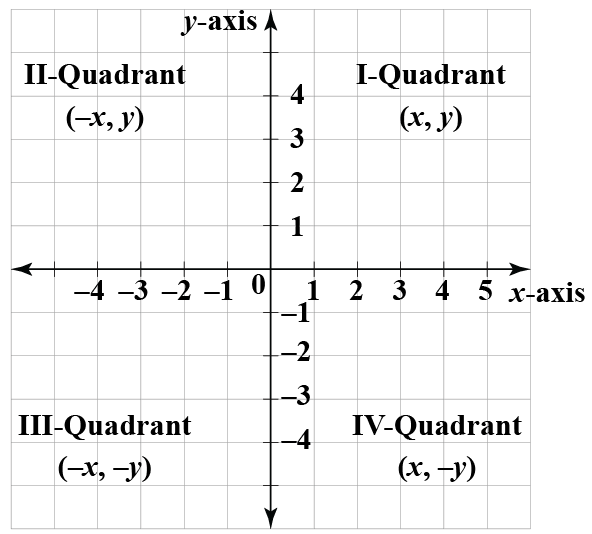The features of the coordinate plane are:

• The plane consists of a horizontal axis called the $$x$$-axis, and a vertical axis called the $$y$$-axis.
• The point of intersection of $$x$$-axis and $$y$$-axis is the origin.
• The coordinates of the origin are $$(0, 0)$$.
• The axis divides the plane into four quadrants

## What Is the Difference Between Scalars and Vectors?

Scalars are simple numeric quantities that are represented on a unidimensional number line.

Vectors are the quantities with both numeric value and direction.

Vectors are generally represented as $$a \hat i + b \hat j$$.

Here $$a$$ and $$b$$ are the number values and $$\hat i$$ and $$\hat j$$ are the unit vectors representing the directions along the $$x$$ axis and $$y$$ axis respectively.

### Examples

• The marks of a student, the currency value, or the volume of a liquid can all be referred to as scalar quantities.
• The variable x = 3 is a scalar quantity.
• The speed of an aircraft has both a numeric value and also the direction associated with it and hence it can be called a vector.
• Also,  $$\bar A = 3 \hat i + 4 \hat j + 5 \hat k$$ represents a vector in maths.

## Equation and Slope of a Line

The slope of a line inclined at an angle $$\theta$$ with the positive $$x$$-axis is:

 $$m = \tan\theta$$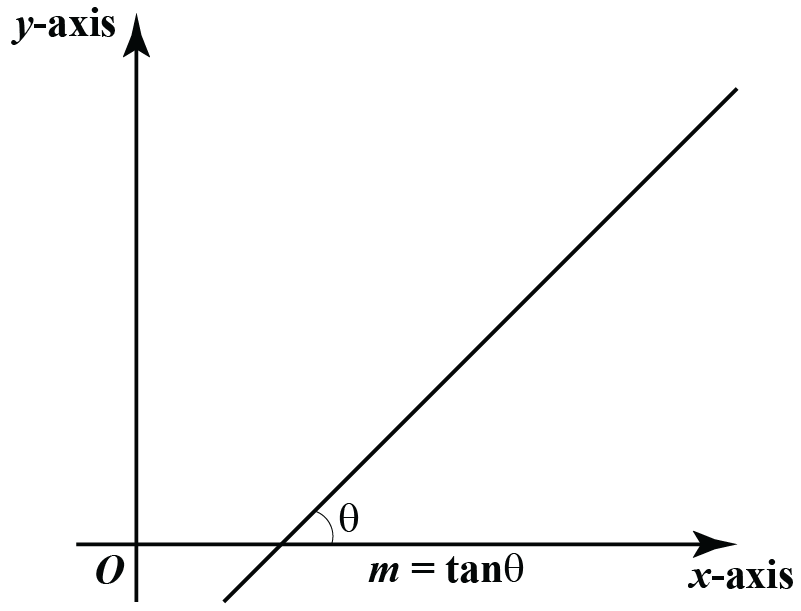The slope of a line passing through the two points $$(x_1, y_1)$$ and $$(x_2, y_2)$$ is:

 $$m = \dfrac{y_2 - y_1}{x_2 - x_1}$$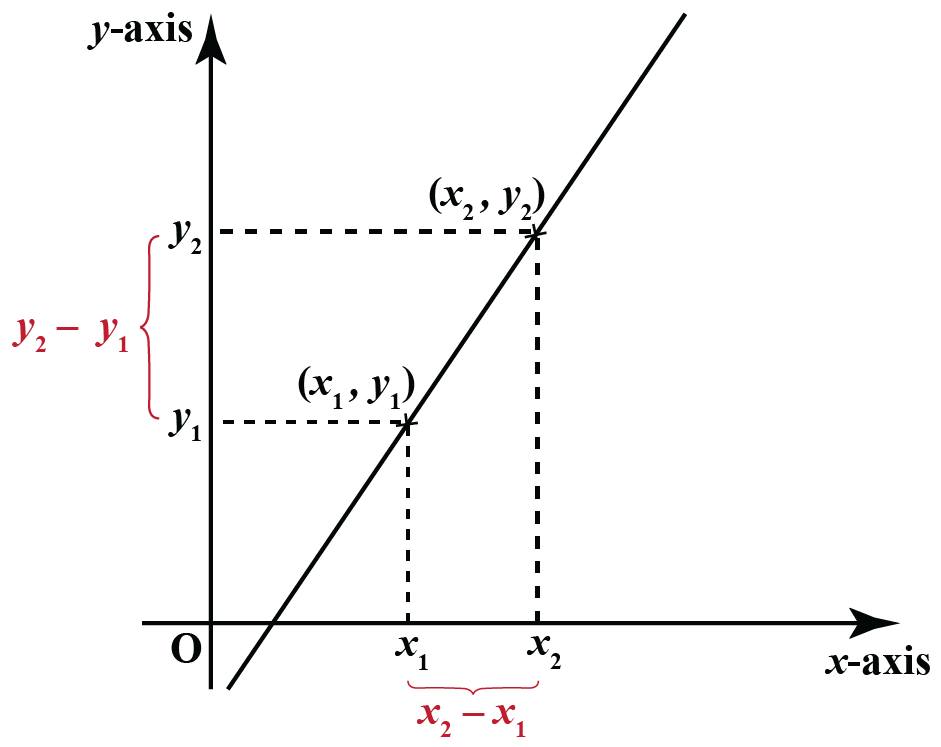The equation of a line passing through the point$$(x_1, y_1)$$ and having a slope m is:

 $(y - y_1) = m(x - x_1)$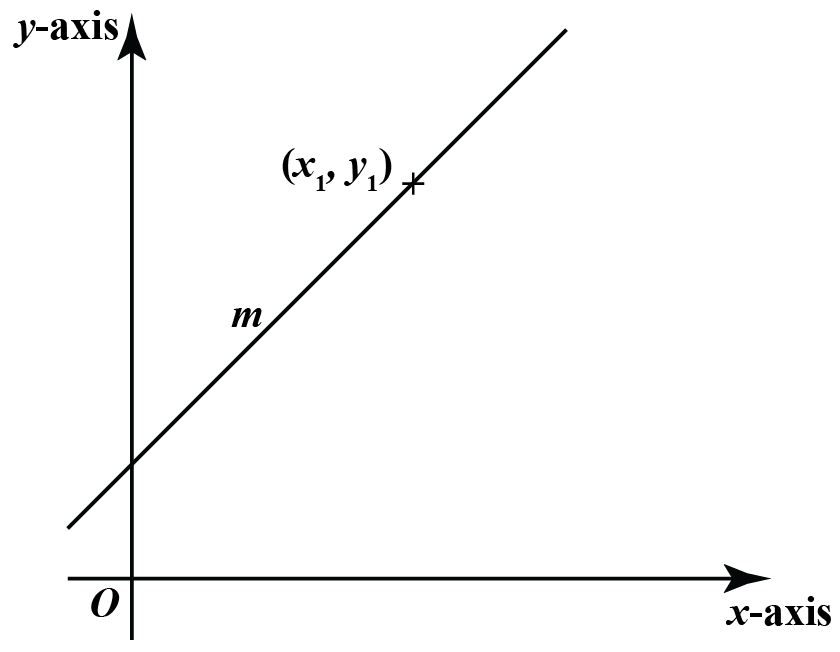The equation of a line passing through the points $$(x_1, y_1)$$ and $$(x_2, y_2)$$ is:

 $(y - y_1) = \frac{(y_2 - y_1)}{(x_2 - x_1)}.(x - x_1)$

The equation of a line cutting the $$y$$-axis at the point $$(0, c)$$ and having a slope $$m$$ is:

 $y = mx + c$

This equation is referred to as the slope-intercept form of equation of a line.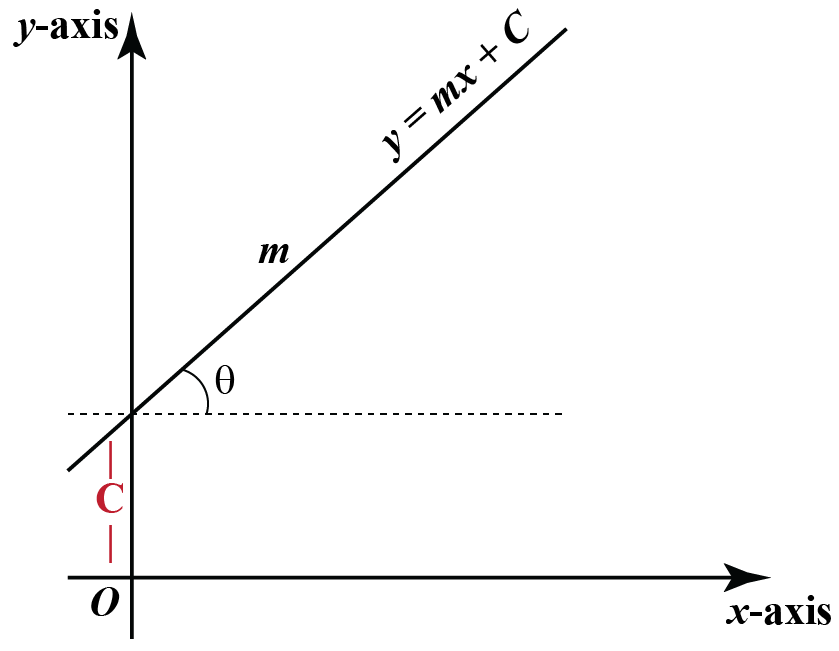## Distance Formula

The distance between two points $$(x_1, y_1)$$ and $$x_2, y_2)$$ is as follows:

 $D = \sqrt{(x_2 - x_1)^2 + (y_2 - y_1)^2}$

### Example

Find the distance between the points $$(-2, 2)$$ and $$(7, -4)$$.

Solution:

The coordinates of the given points can be represented as follows:

$$(x_1, y_1)$$ = (-2, 2) and $$(x_2, y_2)$$ = (7, -4)

Using the distance formula we can find the distance between these points.

\begin{align}d &= \sqrt{(x_2 - x_1)^2 + (y_2 - y_1)^2 }\\ &= \sqrt {(7 - (-2))^2 + (-4 - 2)^2 }\\ &=\sqrt {9^2 +(- 6)^2} \\ &= \sqrt{81 + 36} \\ &= \sqrt {117 }\end{align}

Therefore the distance between the two points is $$\sqrt {117}$$ units.

## Section Formula

The section formulae is useful to find the coordinates of a point which divided the join of the points $$(x_1, y_1)$$ and $$(x_2, y_2)$$ in the ratio $$m : n$$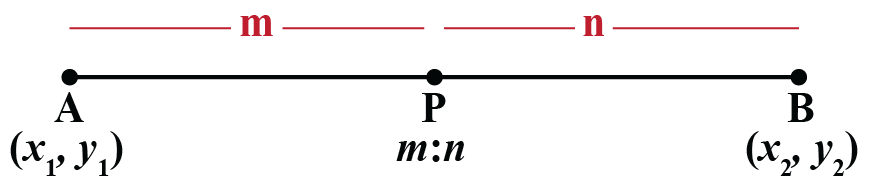$(x, y) = \left(\frac{mx_2 + nx_1}{m + n}, \frac{my_2 + ny_1}{m + n}\right)$

### Example

Find the point which divides the line joining the points $$(-5, 4)$$ and $$(2, 3)$$ in the ratio $$2 : 3$$

Solution:

The coordinates of the given points can be represented as follows.

$$(x_1, y_1) = (-5, 4)$$, $$(x_2, y_2) = (2, 3)$$ and $$m : n = 2 : 3$$

Here we apply the section formula for the given points.

\begin{align} (x, y) &= \left (\dfrac{mx_2 + nx_1}{m + n}, \dfrac{my_2 + ny_1}{m + n}\right) \\ &= \left(\dfrac{2(2) + 3(-5)}{2 + 3}, \dfrac{2(3) + 3(4)}{2 + 3}\right) \\ &=\left(\dfrac{4 -15}{5}, \dfrac{6 + 12}{5}\right) \\ &=\left(\dfrac{-11}{5}, \dfrac{18}{5}\right)\end{align}

Therefore, the point which divides the line joining the two points in the given ratio is $$\left(\dfrac{-11}{5}, \dfrac{18}{5}\right)$$.

## Midpoint Formula

The formula to find the midpoint of the line joining the points  $$(x_1, y_1)$$ and $$x_2, y_2)$$ is as follows.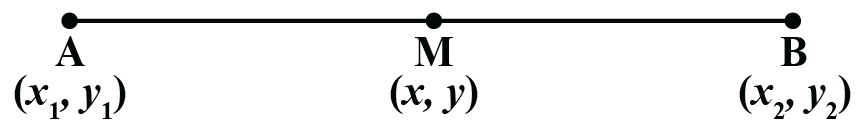$(x, y) = \left(\dfrac{x_1 + x_2}{2}, \dfrac{y_1 + y_2}{2}\right)$

### Example

Find the midpoint of the line joining the points $$(-2, 7)$$ and $$(8, 3)$$

Solution:  Applying the notation for the given points we have $$(x_1, y_1) = (-2, 7)$$, and $$(x_2, y_2) = (8, 3)$$

Here we can apply the midpoint formula.

\begin{align}(x, y) &=\left (\dfrac{x_1 + x_2}{2}, \dfrac{y_1 + y_2}{2}\right)\\&=\left(\dfrac{-2 + 8}{2}, \dfrac{7 + 3}{2}\right)\\&=\left(\dfrac{6}{2}, \dfrac{10}{2}\right)\\&=(3, 4)\end{align}

Therefore the midpoint is $$(3, 4)$$

Coordinate Geometry
Grade 9 | Questions Set 1
Coordinate Geometry
Coordinate Geometry
Grade 10 | Questions Set 1
Coordinate Geometry